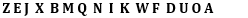# Set-19 Alphanumeric Series For SBI PO and SBI Clerk 2019 | Must Go Through These QuestionsDear Aspirants,
We are providing the most important  Alphanumeric Series Questions for SBI PO 2019, SBI Clerk 2019 and all other competitive bank and insurance exams. These questions have very high chances to be asked in SBI PO 2019, SBI Clerk 2019.
Get the Best Test Series for SBI PO 2019 at the most affordable price (Based on Real Exam Pattern) – Click Here
Download the Best GK Gaming App for Current Affairs and GK (Bank+SSC)– Click here (App No 1)       (App No 2)

Directions (1-5): Study the following series carefully and answer the questions given below.

Z 4 E & J 5 X 2 B M Q 4 8 N 1 I \$ K 7 W F 6 D 9 U # 2 O 3 A

1. Four of the following five are alike in a certain way based on their positions in the above arrangement and so form a group. Which is the one that does not belong to that group?

2. How many such vowels are there in the above arrangement, each of which is immediately preceded by a number and immediately followed by a symbol?

3. How many such numbers are there in the above arrangement, each of which is immediately preceded by a consonant but immediately followed by a number?

4. Which element is at fifth position to the left of the one which is eighth from the right end?

5. If all the numbers and the symbols in the above arrangement are dropped, which of the following element will be the sixth to the left of ‘W’?

Directions (6-10): Answer the following questions referring to the symbol-letter-number sequence given below:

W E & 5 S Q 1 \$ P @ O B 3 V 7 K N U 4 L 8 M 9 % F 6 I G
6. Which of the following is the eleventh element to the right of the second element from the left end in the above sequence?

7. Which of the following is exactly midway between the tenth element from the right and fifth element from the left end?

8. How many letters are there in the above sequence which are immediately preceded by a number and immediately followed by a consonant?

9. What should come in place of the question mark (?) in the following sequence?
W&S, \$@B, 7N4, ?.

10. If both the halves of the above sequence are written in reverse order, which will be the sixth element to the right of the sixteenth element from the right end?

Check your Answers below:

Directions (1-5): Study the following series carefully and answer the questions given below.

Z 4 E & J 5 X 2 B M Q 4 8 N 1 I \$ K 7 W F 6 D 9 U # 2 O 3 A

##### 1. Question

Four of the following five are alike in a certain way based on their positions in the above arrangement and so form a group. Which is the one that does not belong to that group?

Ans: 4
DU6

##### 2. Question

How many such vowels are there in the above arrangement, each of which is immediately preceded by a number and immediately followed by a symbol?

Ans: 1
4 E &, 1 I \$, 9 U #

##### 3. Question

How many such numbers are there in the above arrangement, each of which is immediately preceded by a consonant but immediately followed by a number?

Ans: 4
Q 4 8

##### 4. Question

Which element is at fifth position to the left of the one which is eighth from the right end?

Ans: 3
Thirteenth element from the right is ‘K’.

##### 5. Question

If all the numbers and the symbols in the above arrangement are dropped, which of the following element will be the sixth to the left of ‘W’?

Ans: 1Sixth to the left of W is ‘B’.

Directions (6-10): Answer the following questions referring to the symbol-letter-number sequence given below:

W E & 5 S Q 1 \$ P @ O B 3 V 7 K N U 4 L 8 M 9 % F 6 I G
##### 6. Question
Which of the following is the eleventh element to the right of the second element from the left end in the above sequence?
Ans: 1
##### 7. Question
Which of the following is exactly midway between the tenth element from the right and fifth element from the left end?
Ans: 2
##### 8. Question

How many letters are there in the above sequence which are immediately preceded by a number and immediately followed by a consonant?

Ans: 4
##### 9. Question

What should come in place of the question mark (?) in the following sequence?
W&S, \$@B, 7N4, ?.

Ans: 3
##### 10. Question
If both the halves of the above sequence are written in reverse order, which will be the sixth element to the right of the sixteenth element from the right end?
Ans: 2#### About Study Virus

View all posts by Study Virus →

This site uses Akismet to reduce spam. Learn how your comment data is processed.